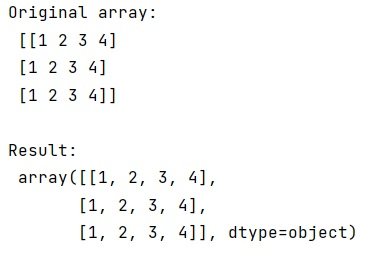# String representation of a numpy array with commas separating its elements

Learn how to represent string of a numpy array with commas separating its elements?
Submitted by Pranit Sharma, on February 10, 2023

NumPy is an abbreviated form of Numerical Python. It is used for different types of scientific operations in python. Numpy is a vast library in python which is used for almost every kind of scientific or mathematical operation. It is itself an array which is a collection of various methods and functions for processing the arrays.

## Representing string of a numpy array with commas separating its elements

Suppose that we are given a NumPy array that contains integer values which we need to convert into string type values and print this array in such a way that each string is separated with a comma.

The string is a group of characters, these characters may consist of all the lower case, upper case, and special characters present on the keyboard of a computer system. A string is a data type and the number of characters in a string is known as the length of the string.

For this purpose, we will use the repr() method while printing the string type array. The repr() method returns a printable representation of the given object.

Let us understand with the help of an example,

## Python code to represent string of a numpy array with commas separating its elements

```# Import numpy
import numpy as np

# Creating a numpy array
arr =  np.array([[1,2,3,4],
[1,2,3,4],
[1,2,3,4]])

# Display original array
print("Original array:\n",arr,"\n")

# Converting array into str type
arr = arr.astype('O')

# Display array using repr function
print("Result:\n",repr(arr),"\n")
```

Output: